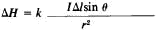# Biot-Savart Law

Also found in: Wikipedia.

## Biot-Savart law

A law of physics which states that the magnetic flux density (magnetic induction) near a long, straight conductor is directly proportional to the current in the conductor and inversely proportional to the distance from the conductor. The field near a straight conductor can be found by application of Ampère's law. The magnetic flux density near a long, straight conductor is at every point perpendicular to the plane determined by the point and the line of the conductor. Therefore, the lines of induction are circles with their centers at the conductor. Furthermore, each line of induction is a closed line. This observation concerning flux about a straight conductor may be generalized to include lines of induction due to a conductor of any shape by the statement that every line of induction forms a closed path.

## Biot-Savart Law

a law that determines the strength of the magnetic field created by an electric current. The Biot-Savart law was discovered by the French scientists J. B. Biot and F. Savart in 1820 and given a general formulation by P. Laplace. According to this law, a small segment of a conductor Δl along which a current of strength / is flowing creates—at a given point M in space, located at a distance r from the segment Δl (Δl << r)—a magnetic field of strengthHere θ is the angle between the direction of the current in segment / and the radius vector r, drawn from the segment to the observation point M, and k is the coefficient of proportionality, which depends on the choice of the system of units. In the centimeter-gram-second (cgs) system (Gauss), k=1/c, where c = 3 x 1010 cm/sec (the velocity of light in a vacuum); in the International System of Units (SI), k = l/4π.

The magnetic field strength ΔH is perpendicular to the plane P, which contains Δl and r, and its direction is determined by the auger rule: if the shaft of the auger (with a right-handed rifling) is turned from Δl toward r, then the auger advances in the direction of ΔH.

The total magnetic field strength ΔH created by a conductor with a current at point M is equal to the vector sum of the quantities ΔH, which are determined by all the elements Δ/ of the conductor. In particular, the strength H of a magnetic field at a distance d from a long (much greater than d), straight conducting wire along which a current of strength / is flowing is H = k·2I/d; in the center of a circle with radius R along which a current of strength / is flowing, H = k. 2πI/R; and on its axis, at a point remote from the plane of the circle at a distance d ≪ R, H = k·2π R2I/d3. On the axis of a solenoid of n turns, H = k·4πn/.

The Biot-Savart law can also be considered as a law that determines the magnetic induction ΔB ; in the cgs system, this requires that the expression for ΔH be multiplied by the magnetic permeability of the medium μ, whereas in the SI system, in addition, it must be multiplied by the magnetic permeability of a vacuum, μo = 4π10-7 henry/m.

G. IA. MIAKISHEV

## Biot-Savart law

[¦byō sə¦vär ′lȯ]
(electromagnetism)
A law that gives the intensity of the magnetic field due to a wire carrying a constant electric current.
Mentioned in ?
References in periodicals archive ?
In this section, Prandtl's classical lifting-line theory for a single finite wing is presented firstly, and then, the lifting-line theory for tandem wing configuration is proposed on the foundation of Prandtl's classical lifting-line theory combined with the Biot-Savart law.
The flux density generated by the PMG alone and calculated with Biot-Savart law or static FEM is used as excitation field in the finite element method [1,18,21-23].
The ECL also leverages the Biot-Savart Law, which describes the relationship between a steady line current source and magnetic flux density at a point.
Den, "A new method using Biot-Savart law to derive magnetic scalar potential notation," Journal of Chongqing Institute of Civil Engineering and Architecture, Vol.
Due to this, to obtain the reliable magnetic induction calculation, technique based on discrete approximation of the Biot-Savart law is chosen.
The magnetic field is determined taking into account the catenary equation and applying the Biot-Savart law. In appendix of  is mentioned that expressions containing hyperbolic functions, as is the case with their obtained expressions, often cannot be integrated analytically.
The derivation of this relation begins with equation for computing of magnetic field B inside single wire loop, which is derived from the Biot-Savart law (Trout, 1988):
Grover starts from the basic Biot-Savart Law, which comes from Ampere's Law and Gauss's Law, each, one of Maxwell's equations, and derives all his approximations.
These coils should be smooth and should be constructed by a filtered form of the Biot-Savart law to generate an external magnetic field, consistent with the one defined by current inside the plasma, that has robust flux surfaces devoid of extraneous harmonics (1).
The magnetic field due to a single moving charged particle (q) is given by the Biot-Savart law as
We estimate the tensor-product B-spline coefficients from values of |B| computed on a grid by a numerical code that numerically solves the Biot-Savart law numerically corresponding to the geometry of the solenoid and current bars that produce the magnetic field.

Site: Follow: Share:
Open / Close